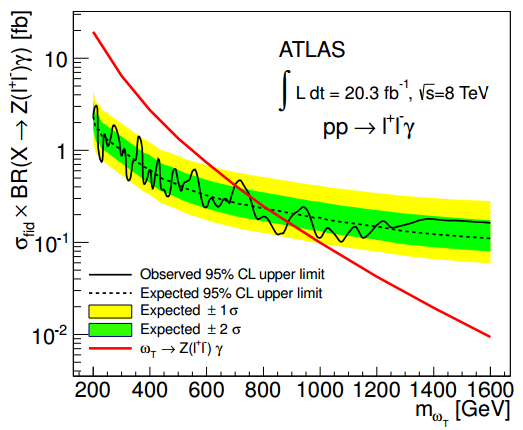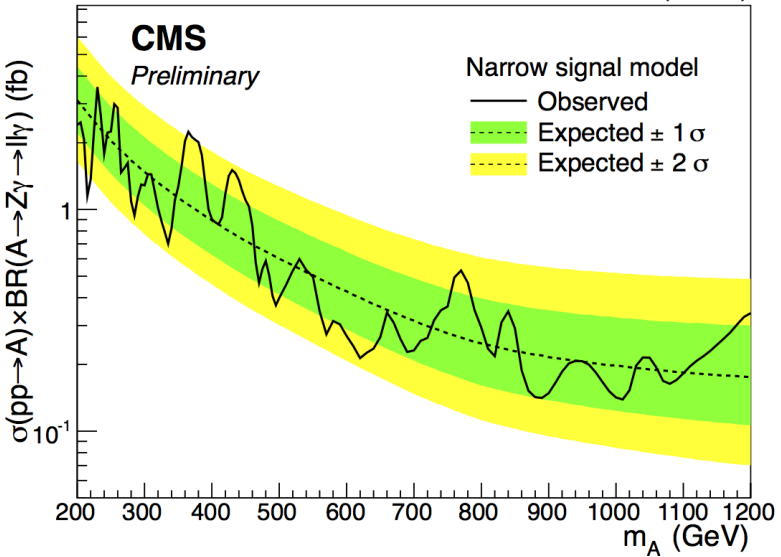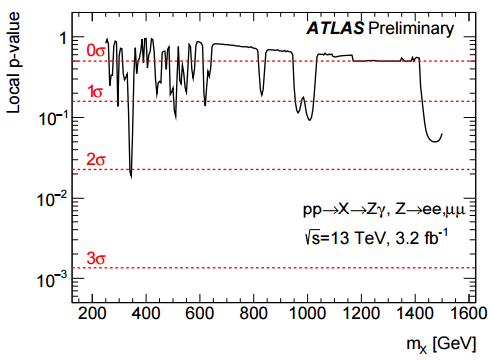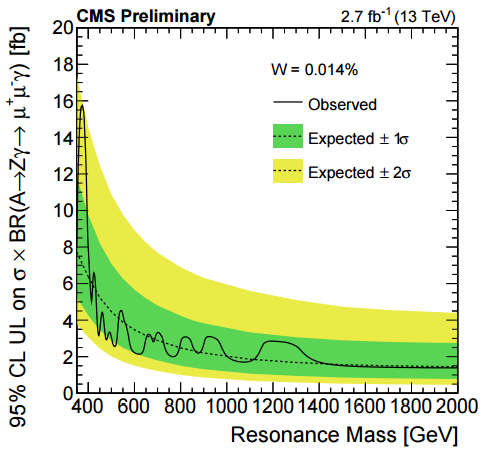## Monday, March 28, 2016

### $$Z\gamma$$: excess near $$375\GeV$$ in new and old ATLAS and CMS data

Happy Easter!

I hope that your Jesus has been resuscitated as in every year and the Israeli readers have passed over whatever they had to. Czechs shouldn't forget that we're living in a leap year. According to some (but not all) authorities, because it's a leap year in 2016, girls should whip the boys today instead of the prevailing setup (a way to guarantee an equality of sexes). Ladies and gentlemen, it's a femdom Easter. ;-)

The music is from Nightwork's hit In sweatpants, move your balls to the right side.

But Easter Monday is just another day in experimental particle physics and I just finally accumulated all the four relevant graphs in a channel... and they seem to suggest something very interesting.

There are two universal detectors to detect new physics at the LHC: ATLAS and CMS. They have a different design and two different teams work on them. This split may be presented as a great virtue. The nice ATLAS folks can independently verify the claims by the jerks at CMS, and vice versa. However, this split may also be considered a giant waste of time and data. In some sense, the artificial split of the collisions into two sets reduces all the significance levels in all articles by a factor of $$\sqrt{2}$$.

OK, I believe that there should exist professional particle physicists who simply ignore the split and who are trying to construct the ATLAS+CMS combinations as if the split didn't exist.

Without these professionals, people have to do it informally. Let me show you the four relevant graphs with the $$Z\gamma$$ final state where the $$Z$$-boson decays to a charged lepton pair $$\ell^+\ell^-$$. This channel is interesting because ATLAS and CMS are observing a strong but inconclusive excess in the diphoton $$\gamma\gamma$$ channel near $$M=750\GeV$$. Every particle decaying to $$\gamma\gamma$$ should be able to decay to $$Z\gamma$$ as well because both $$Z$$ and $$\gamma$$ arise as "parts" of gauge vector pure states that are more natural in high-energy fundamental theories, namely $$B^0$$ and $$W^0$$ gauge bosons of $$U(1)$$ and $$SU(3)_z$$ of the electroweak theory.

The excess near $$750\GeV$$ isn't clearly seen in the $$Z\gamma$$ papers so far, I think. However, it seems to me that all four papers about $$Z\gamma$$ seem to suggest an excess rather close to $$375\GeV$$ or so which just happens to be one-half of $$750\GeV$$. In the following graphs, "old" and "new" means 2012 and 2015 (i.e. $$8\TeV$$ and $$13\TeV$$), respectively.ATLAS old, Figure 3b. There are many wiggles but the marginally strongest upward fluke seems to be close to $$M=375\GeV$$.CMS old, Figure 2. The strongest excess is broader here but it is close to the mass $$M=375\GeV$$, too.ATLAS new, Figure 4a. The strongest excess is around $$M\sim 340\GeV$$ which is a bit lower than the previous two but it might be close enough.CMS new, Figure 3 upper right. This picture captures only events where the $$Z$$ decays to a charged muon pair, with the assumed low width of the particle. The mass of the highest excess is very close to $$M\sim 375\GeV$$ again. Note that the electron pair picture has a local excess near $$M\sim 620\GeV$$.

Now, the appearance of a very similar mass of the excess in all four papers seems a bit intriguing to me. I am making several speculations here. First, there could be a new particle or new particles with the invariant mass $$M\sim 375\GeV$$ that decays to $$Z\gamma\to \ell^+\ell^-\gamma$$.

Second, this $$375\GeV$$ could be one-half of $$750\GeV$$ and there could be a reason for those things. Maybe the object near $$750\GeV$$ is actually composed of two particles of the same type (or particle-antiparticle pair) near a threshold, perhaps a bound state. My knowledge would indicate that the $$Z\gamma$$ and $$\gamma\gamma$$ decays should show pretty much the same in that case. But maybe there exists a reason (some discrete conservation law?) why the $$Z\gamma$$ decays may already arise from a single copy of this new particle while the $$\gamma\gamma$$ channel (which has the symmetry between the two particles in the final state) seems to demand two copies of the new particle.

I am going to think about possible models that could imply such a thing. Recall that the ATLAS diphoton graph also shows an excess near $$1.6\TeV$$, close to two times $$750\GeV$$, and $$375\GeV$$ is just a bit higher than twice the top quark mass, $$173\GeV$$. These resonances with the multiplicative spacings given by the factors of two almost look like some of the "spectra of the Riemann Hypothesis and primes" I am playing with at other moments.

Ivan Mládek, A Sourball's (Global Warming Alarmist's) Spring Song. Lyrics: A sourball with a flute is playing a song about the spring, and then, as is commonplace in music, after his flute solo, he also sings: The damn spring has arrived again, birds yell and the lilacs stink. Because of all those chickens, there's no place to spit, in the warm weather, my dried honey has soured. The Sun is annoyingly creeping into the villa, my snowman is already collapsing. Ices are cracking and the baulks are drying up, Annie is painfully twisting her body. Because of an Easter whip, kids are ruining a willow. Goslings are sizlling like snakes at the doorstep. The damn spring is already here again, I prefer not to approach the window. Again, the buds, blossoms, and grass, I will once get mad in the spring.